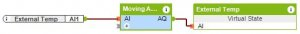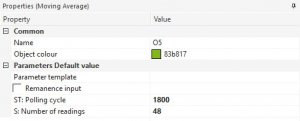# Moving Average

## FUNCTIONS

Connect the analogue value that you want to take a moving average of (for example a temperature sensor) to the input AI. ST sets how often the input value is read. Using the parameter S you can set how many readings that are averaged every ST seconds.

The average is then output at AQ.

#### EXAMPLEThe analogue value is an external temperature sensor and is connected to AI. Every 1800 seconds (parameter ST) the input value is read.

The average is then calculated using the last 48 values (parameter S).

The calculated mean value every 30 minutes is the average external temperature over the last 24 hours.

This is because every 30 minutes an average is taken and there are 48 values used in this average (which is therefore 2 values per hour equalling in a 24-hour average). The same 24-hour average could be achieved taking a reading every 3600 seconds (ST) with 24 readings (S) used in the average.Example of use:

Ventilation control, climate control, heating control, monitoring etc.